Double Entry Book Keeping Ts Grewal (2017) Solutions for Class 12 Commerce Accountancy Chapter 2 Goodwill: Concept And Mode Of Valuation are provided here with simple step-by-step explanations. These solutions for Goodwill: Concept And Mode Of Valuation are extremely popular among class 12 Commerce students for Accountancy Goodwill: Concept And Mode Of Valuation Solutions come handy for quickly completing your homework and preparing for exams. All questions and answers from the Double Entry Book Keeping Ts Grewal (2017) Book of class 12 Commerce Accountancy Chapter 2 are provided here for you for free. You will also love the ad-free experience on Meritnation’s Double Entry Book Keeping Ts Grewal (2017) Solutions. All Double Entry Book Keeping Ts Grewal (2017) Solutions for class 12 Commerce Accountancy are prepared by experts and are 100% accurate.

#### Answer:Number of years’ purchase = 3#### Answer:

Calculation of average profits for the last three years

Calculation of average profits for the last four years

Average Profits for last four years is higher than the Average Profits for last three years. Thus, Goodwill of the firm is 2,27,550.

#### Answer:

 Year Profit 2013-14 8,00,000 2012-13 15,00,000 2011-12 18,00,000 2010-11 (4,00,000) 2009-10 13,00,000 Total Profit 50,00,000

Goodwill = Avg. Profits $×$ No. of Years' Purchase
= 10,00,000 $×$ 3
= Rs 30,00,000

#### Answer:

Calculation of Average Profit
2012–13 = 1,00,000 – 12,500 = 87,500

2013–14 = 1,25,000 + 25,000 = 1,50,000

2014–15 = 1,12500 – 12,500 = 1,00,000

Total Profits for last three years = 3,37,500

#### Answer:

 Year 2009–10 2010–11 2011–12 2012–13 2013–14 Profit/Loss 1,50,000 3,50,000 5,00,000 7,00,000 (6,00,000) Add: Wrong Debit 1,00,000 Less: Depreciation (25,000) Total 1,50,000 3,50,000 5,00,000 7,00,000 (5,25,000)

#### Answer:

Goodwill = Normal Average Profit × Number of years' purchase

 Year Actual Profit + Abnormal Loss Non-recurring – Abnormal Gain Non-recurring = Normal Profit 2017 30,000 + 40,000 – Nil = 70,000 2016 (80,000) + 1,10,000 – Nil = 30,000 2015 1,10,000 + Nil – 30,000 = 80,000 Normal Profit for 3 Years 1,80,000Number of years’ purchase is 2Goodwill = 60,000 × 2 = Rs 1,20,000

#### Answer:Year Actual Profit + Abnormal Loss Non-recurring – Abnormal Gain Non-recurring = Normal Profit 2015 50,000 + Nil – 5,000 = 45,000 2016 (20,000) + 30,000 – Nil = 10,000 2017 70,000 + Nil – 18,000+8,000 = 44,000 Normal Profit for 3 Years 99,000Number of years’ purchase = 2#### Answer:

Working Notes:

WN: 1 Calculation of Normal Profits

 Year Profit/(Loss) (Rs) Adjustment Normal Profit (Rs) 31 March, 2014 80,000 20,000 1,00,000 31 March, 2015 1,45,000 (25,000) 1,20,000 31 March, 2016 1,60,000 (15,000) 1,45,000 31 March, 2017 2,00,000 2,00,000 5,45,000

WN: 2 Calculation of Average Profit

#### Answer:

Working Notes:

WN: 1 Calculation of Normal Profits

 Year Profit/(Loss) (Rs) Adjustment Normal Profit (Rs) 31 March, 2013 (90,000) - (90,000) 31 March, 2014 1,60,000 (50,000) 1,10,000 31 March, 2015 1,50,000 20,000 1,70,000 31 March, 2016 65,000 85,000* 1,50,000 31 March, 2017 1,77,000 (17,000) 1,60,000 5,00,000

* Adjustment Amount

 Overhauling cost of second hand machinery wrongly accounted as expense instead of capital expenditure. Profit to be increase by Rs 1,00,000 1,00,000 Depreciation to be debited from P&L A/c $\left(1,00,000×\frac{20}{100}×\frac{9}{12}\right)$ (15,000) Amount to be added back 85,000

WN: 2 Calculation of Average Profit

#### Answer:

 Year Profit × Weight = Product 2013 20,000 × 1 = 20,000 2014 24,000 × 2 = 48,000 2015 30,000 × 3 = 90,000 2016 25,000 × 4 = 1,00,000 2017 18,000 × 5 = 90,000 Total 15 3,48,000#### Answer:

 Year Profit before Partners’ Remuneration – Partners’ Remuneration = Profit after Partners’ Remuneration 2014-15 2,00,000 – 90,000 = 1,10,000 2015-16 2,30,000 – 90,000 = 1,40,000 2016-17 2,50,000 – 90,000 = 1,60,000

 Year Profit × Weight = Product 2014-15 1,10,000 × 1 = 1,10,000 2015-16 1,40,000 × 2 = 2,80,000 2016-17 1,60,000 × 3 = 4,80,000 Total 6 8,70,000#### Answer:

Goodwill = Weighted Average Profit × No. of years purchase

 Year Profit before Salary Salary Profit after Salary Weights Weighted Profit A B C = A – B D E = C × D 2012 1,40,000 90,000 50,000 1 50,000 2013 1,01,000 90,000 11,000 2 22,000 2014 1,30,000 90,000 40,000 3 1,20,000 Total 6 1,92,000

#### Answer:

Calculation of Normal Profit

 Year Particulars Normal Profit 2005 25,000 - 5000 (Management Cost) = 20,000 2006 27,000 + 10,000 (Plant Repair) – 1,000 (Deprecation) – 1000 (Closing Stock)  - 5000 (Management Cost) = 30,000 2007 46,900 – 900 (Deprecation) +1,000 (Opening Stock) – 2,000 (Closing Stock) – 5,000 (Management Cost) = 40,000 2008 53,810 – 810 (Deprecation) + 2,000 (Opening Stock) – 5,000 (Management Cost) = 50,000

Calculation of Weighted Profit

 Year Normal Profit × Weight = Product 2005 20,000 × 1 = 20,000 2006 30,000 × 2 = 60,000 2007 40,000 × 3 = 1,20,000 2008 50,000 × 4 = 2,00,000 Total 10 4,00,000#### Answer:

 Year Profit × Weight = Product 2013 45,000 × 1 = 45,000 2014 15,000 × 2 = 30,000 2015 44,000 × 3 = 1,32,000 Total 6 2,07,000

Weighted Average Profit

Goodwill = Weighted Average Profit × Number of Years’ Purchase

= 34,500 × 2 = Rs 69,000

Goodwill brought in by Z = Total Goodwill × His share of Profit

Working Note:

Profits for the past years:

 Year Profit Abnormal Profit Abnormal Loss = Profit 2013 50,000 $-$ 5,000 = 45,000 2014 (20,000) + 35,000 = 15,000 2015 70,000 - 26,000* = 44,000 Total 2,07,000

*Total Abnormal Gain = 18,000 (Insurance claim received) + 8,000 (Interest & Dividend received) = 26,000

#### Answer:

Working Notes:

WN: 1 Calculation of Normal Profits:

 Year Profit/(Loss) (Rs) Adjustment Normal Profit (Rs) 31 March, 2013 70,000 20,000 90,000 31 March, 2014 1,40,000 (30,000) 1,10,000 31 March, 2015 1,00,000 - 1,00,000 31 March, 2016 1,60,000 (10,000) 1,50,000 31 March, 2017 1,65,000 10,000 1,75,000

WN: 2 Calculations of Weighted Average Profits:

 Year Normal Profit Weight Product 31 March, 2013 90,000 1 90,000 31 March, 2014 1,10,000 2 2,20,000 31 March, 2015 1,00,000 3 3,00,000 31 March, 2016 1,50,000 4 6,00,000 31 March, 2017 1,75,000 5 8,75,000 Total 15 20,85,000

#### Answer:

Working Notes:

WN: 1 Calculation of Normal Profits:

 Year Profit/(Loss) (Rs) Adjustment Normal Profit (Rs) 31 March, 2013 1,25,000 - 1,25,000 31 March, 2014 1,40,000 - 1,40,000 31 March, 2015 1,20,000 - 1,20,000 31 March, 2016 55,000 1,35,000* 1,50,000 31 March, 2017 2,57,000 (67,000)** 1,90,000

* Adjustment Amount

 (1) Amount spent at the time of purchase of machinery wrongly accounted as expense instead of capital expenditure. Profit to be increase by Rs 1,00,000 1,00,000 Depreciation to be debited from P&L A/c $\left(1,00,000×\frac{20}{100}×\frac{9}{12}\right)$ (15,000) Amount to be added back 85,000 (2) Closing stock being undervalued on 31st March, 2016 means profit is shown at lower profit.

** Adjustment Amount

 (1) Written down value as on 1st April, 2016 on amount spent 85,000 Depreciation to be debited from P&L A/c $\left(85,000×\frac{20}{100}\right)$ (17,000) Amount to be added back 68,000 (2) Closing stock being undervalued on 31st March, 2016 means profit is shown at lower profit. Profit for next year shown at higher amount as closing stock of previous year is carried forward as opening stock of next year.

WN: 2 Calculations of Weighted Average Profits:

 Year Normal Profit Weights Product 31 March, 2013 1,25,000 1 1,25,000 31 March, 2014 1,40,000 2 2,80,000 31 March, 2015 1,20,000 3 3,60,000 31 March, 2016 1,90,000 4 7,60,000 31 March, 2017 1,90,000 5 9,50,000 Total 15 24,75,000

#### Answer:

Working Notes:

WN1: Calculation of Future Maintainable Profits

#### Answer:Number of years’ purchase = 4#### Answer:Number of years’ purchase = 3#### Answer:Number of years’ purchase = 2#### Answer:Number of years’ purchase = 2#### Answer:Year Profit before Partners’ Remuneration – Partners’ Remuneration = Actual Profit after Remuneration 2013–14 1,70,000 – 1,00,000 = 70,000 2014–15 2,00,000 – 1,00,000 = 1,00,000 2015–16 2,30,000 – 1,00,000 = 1,30,000Number of years’ purchase = 2#### Answer:#### Answer:Number of years’ purchase = 4#### Answer:Capital Employed = Total Assets Creditors

= 75,000 5,000 = Rs 70,000Goodwill of the firm = Rs 24,000

Number of years’ purchase = 4Or, 24,000 = Super Profit × 4#### Answer:

Working Notes
:

WN1: Calculation of Future Maintainable Profits

#### Answer:

Average Profit earned by a firm = Rs 7,50,000
Overvaluation of Stock = Rs 30,000
Average Actual Profit = Average Profit earned by a firm – Overvaluation of Stock
or, Average Actual Profit = 7,50,000 – 30,000 = Rs 7,20,000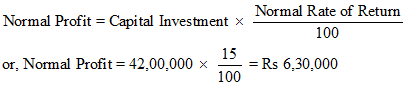Super Profit = Actual Average Profit – Normal Profit
or, Super Profit = 7,20,000 – 6,30,000 = Rs 90,000
Goodwill = Super Profit × Number of Times
Goodwill = 90,000 × 3 = Rs 2,70,000

#### Answer:

Working Notes:

WN: 1 Calculation of Normal Profits:

 Year Profit/(Loss) (Rs) Adjustment Normal Profit (Rs) 31 March, 2013 1,50,000 - 1,50,000 31 March, 2014 1,80,000 - 1,80,000 31 March, 2015 1,00,000 1,00,000 2,00,000 31 March, 2016 2,60,000 (40.000) 2,20,000 31 March, 2017 2,40,000 - 2,40,000 Total Profit 9,90,000

WN2: Calculation of Super Profits

WN3: Calculation of Capital Employed

#### Answer:Total Capital = Rs 16,00,000#### Answer:#### Answer:

Capital Employed = Total Tangible Assets – Outside Liabilities

Capital Employed = 28,00,000 – 8,00,000 = Rs 20,00,000

Average Profit = Rs 3,00,000

Super Profit= Average Profit– Normal Profit

Super Profit= 3,00,000 – 2,00,000 = 1,00,000

#### Answer:#### Answer:

Working Notes
:

WN1: Calculation of Future Maintainable Profits

#### Answer:

Working Notes:

WN1: Calculation of Super Profits

WN2: Calculation of Capital Employed

#### Answer:

(i)

(ii)

Working Notes:

WN1: Calculation of Super Profits

#### Answer:

WN1: Calculation of Average and Super Profits

#### Answer:

Average Profit – Rs 4,00,000
Normal Rate of Return – 10%

(i) Goodwill by Capitalisation of Super profit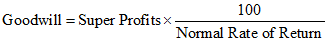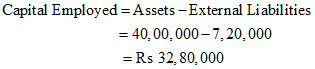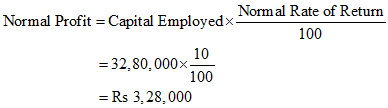Super Profit = Actual Profit – Normal Profit
= 4,00,000 – 3,28,000
= Rs 72,000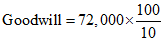Goodwill = Rs 7,20,000

(ii) Super Profit Method if the goodwill is valued at 3 years’ purchase of super profits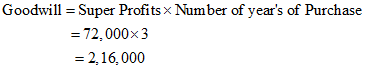Therefore, Goodwill is valued at Rs 2,16,000

#### Answer:

(i) Calculation of goodwill through Capitalisation Method:

Average Capital Employed = 9,00,000 + 6,00,000 = 15,00,000

Goodwill = Capitalised Value of the Firm – Average Capital Employed

Goodwill = 22,50,000 – 15,00,00 = Rs 7,50,000

(ii) Calculation of goodwill through Super Profit Method:

Average Profit = 4,50,000

Super Profit = Average Profit – Normal Profit

Super Profit = 4,50,000 – 3,00,000 = Rs 1,50,000

Goodwill = Super Profit × No. of Years’ purchase

Good will = 1,50,000 × 2 = 3,00,000

#### Answer:

(i) Calculation of Goodwill by Capitalisation of Super Profit MethodProfit of the firm = Rs 5,00,000(ii) Calculation of Goodwill by Capitalisation of Average Profit Method#### Answer:

(i)

(ii)

(iii)

(iv)

View NCERT Solutions for all chapters of Class 15